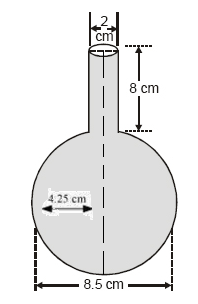Newbie

# A spherical glass vessel has a cylindrical neck 8 cm long, 2 cm in diameter; the diameter of the spherical part is 8.5 cm. By measuring the amount of water it holds, a child finds its volume to be 345 cm3. Check whether she is correct, taking the above as the inside measurements, and π = 3.14.Q.8

• 0

How i solve the ncert class 10 surface areas and volumes  Find the solution of exercise 13.2 question no.8 . Also find the easiest and simplest form of this question . A spherical glass vessel has a cylindrical neck 8 cm long, 2 cm in diameter; the diameter of the spherical part is 8.5 cm. By measuring the amount of water it holds, a child finds its volume to be 345 cm3. Check whether she is correct, taking the above as the inside measurements, and π = 3.14.

Share

1. Given,

For the cylinder part, Height (h) = 8 cm and Radius (R) = (2/2) cm = 1 cm

For the spherical part, Radius (r) = (8.5/2) = 4.25 cmNow, volume of this vessel = Volume of cylinder + Volume of sphere

= π×(1)2×8+(4/3)π(4.25)3

= 346.51 cm3

• 0